# Inscribed Shapes In Circle (Triangles, Squares, & More)

We can inscribe lots of different shapes inside of a circle, including triangles, squares, pentagons, and others.

An inscribed shape inside of a circle is as large as possible while still fitting inside of the circle. The vertices (corners) of the shape will intersect the circle on its circumference. We can calculate the perimeter and area for a shape inscribed in a circle.

Of course, we can also inscribe a circle inside of these shapes (triangles, squares, and so forth), which gives us a different result for perimeter and area.

In this article, we’ll talk about inscribed triangles, squares, and other shapes inside of a circle.  We’ll also learn how to find the perimeter and area of these shapes.

Let’s get started.

## Inscribed Shapes In Circles

When we inscribe a regular polygon (such as an equilateral triangle or a square) in a circle, the shape is “as large as possible” while still fitting inside of the circle.

With a little algebra, we can calculate the dimensions (side lengths, perimeter, and area) for each of these polygons, given the radius of the circle they are inscribed in.

Let’s start with an equilateral triangle inscribed in a circle.

Names of the shapes | Useful List o...
Names of the shapes | Useful List of Geometric Shapes with Images

### Can A Triangle Be Inscribed In A Circle?

We can inscribe a triangle inside of a circle.  One of the simplest cases is an equilateral triangle (with side length S) inscribed in a circle (with radius R), which you can see below.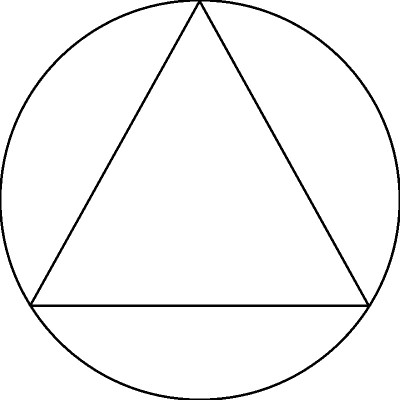Here we see an equilateral triangle inscribed in a circle. Remember that an equilateral triangle has three sides of equal length and three angles of equal measure (all are 60 degrees).

We can label the graph with a few key elements:

• A point C at the center of the circle (and at the center of the equilateral triangle)
• A line segment from each vertex of the triangle to the center point C (each has a length of R)
• The side lengths S of the triangle

You can see the updated picture below.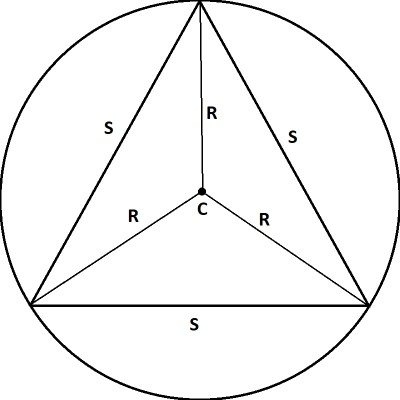Here we have labelled the side lengths of our equilateral triangle as “S”. We have also drawn a center point “C” and line segments from the vertices of the triangle to C (all of these line segments have a length of R, the radius of the circle).

We can also make a few more additions so that we can solve for the side length S of the triangle in terms of the radius R of the circle:

• Since all angles in an equilateral triangle are 60 degrees, and the lines in our diagram bisect those angles, then we can label the smaller angles as 30 degrees each.
• This means that the large central angles must be 120 degrees (since the angles in a triangle add up to 180, and the two small angles are 30 each, for a total of 60, and 180 – 60 = 120).
• Take the vertical line segment from the top of the triangle to the center of the circle and extend it to the bottom of the triangle.
• This bisects the large central angle into two 60 degree angles.
• This also bisects the base S of the triangle into two equal lengths, S/2.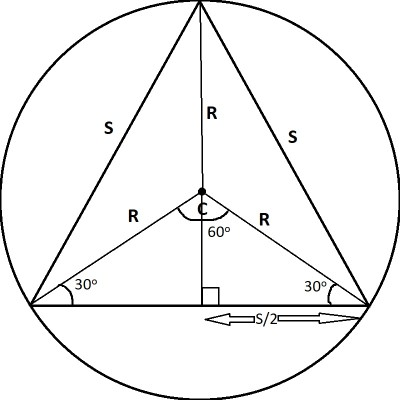We get a special triangle (with angles 30, 60, and 90 degrees), which we can easily solve with proportions and cross multiplication.

Now we can see that we have a special triangle (with angles 30, 60, and 90), with a leg of length S/2 (the base) and a hypotenuse of length R (the radius of the circle).

In a 30-60-90 triangle, the corresponding side lengths have a ratio of 1:√3:2.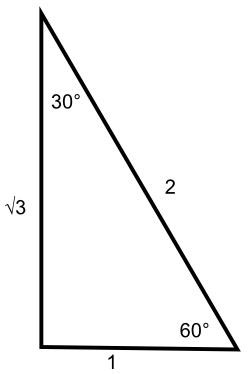A 30-60-90 triangle has side lengths in the ratio 1:√3:2 (the longest side is across from the largest angle).

Here, we can set up a proportion to solve for S:

• √3 / 2 = (S/2) / R  [√3 and S/2 are the sides across from 60 degrees; 2 and R are the hypotenuses]
• √3 / 2 = S/2R  [simplify nested fraction on the right side]
• (√3)*2R = 2S  [cross multiply]
• R√3 = S  [divide both sides by 2]

So, we can see that the side length S of the equilateral triangle is R√3.  Since √3 is approximately 1.73, we know that the side length S is about 73% larger than the radius R of the circle.

This makes sense, since S is the largest side of a triangle whose two other side lengths are R and R (meaning the length of the longest side is between R and 2R).

Alternately, the radius of the circle is the side length of the equilateral triangle divided by √3, or:

• R√3 = S
• R = S/√3  [divide both sides by √3]
• R = S√3/3  [rationalize the denominator]

Since the perimeter of an equilateral triangle is 3S (where S is the side length), then the perimeter of this triangle is 3S = 3(R√3) = (3√3)R, or approximately 5.196 times the length of the radius R of the circle.

Since the area of an equilateral triangle is BH/2 (base times height divided by 2), we need to find the height (we already know the base is S = R√3).

The height is the other leg of the 30-60-90 triangle we used above.  Since the ratio is 1:√3:2, we know that the side across from the 30 degree angle has half the length of the hypotenuse.

Since the height of the triangle is across from the 30 degree angle, then its length is half of the hypotenuse, or R/2.

The height of the entire triangle is R (the top portion of the line segment) plus R/2 (the bottom portion of the line segment), for a total height of 3R/2.

So, the area of the triangle is:

• BH/2
• =(R√3)(3R/2) / 2
• =R23√3/4

This comes out to approximately 1.3R2, which is less than half of the area of the circle, πR2.  This makes sense, since the equilateral triangle is completely contained within the circle.

### Can A Square Be Inscribed In A Circle?

We can inscribe a square inside of a circle.  When you inscribe a square in a circle, you are finding the largest square that can fit inside of that circle.

Another way to think of it is finding the smallest circle that will contain the square. You can see what this looks like in the diagram below.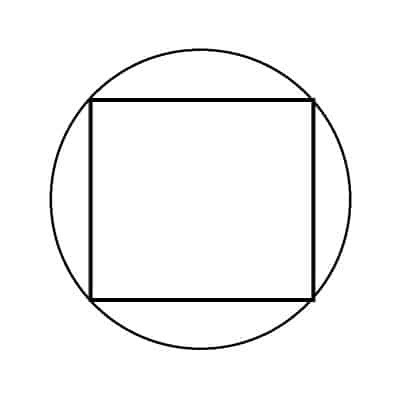Here we see a square inscribed in a circle. Remember that a square has four sides of equal length and four equal angles, all with a measure of 90 degrees.

We would have a square (with side length S) inscribed in a circle (with radius R), which you can see below (the center point C is the center of both the circle and the square).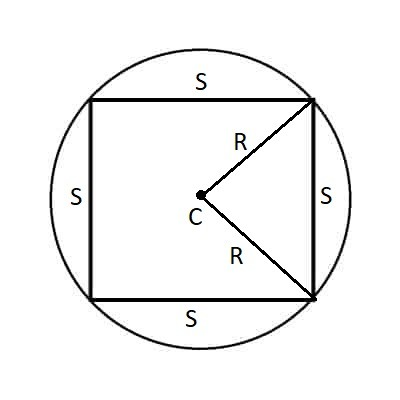We have labelled the side lengths of our square as “S” and drawn a center point “C”. We have also drawn line segments from two vertices of the square to the center point C (each of these line segments has a length of R, the radius of the circle).

Note the following:

• The line segment from each corner of the square to the center point C bisects a 90 degree angle into two 45 degree angles.
• The central angle of the triangle (at point C, where the two radii meet) is a 90 degree angle.

You can see this in the diagram below: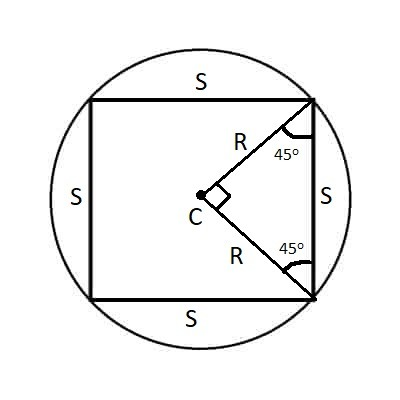The largest angle of the triangle is 90 degrees, and each of the other two angles is 45 degrees, giving us a special triangle that we can solve with ratios and proportions for S.

Now we can see that we have a special triangle (with angles 45, 45, and 90), with legs of length R (the base) and a hypotenuse of length S (the side length of the square).

In a 45-45-90 triangle, the corresponding side lengths have a ratio of 1:1:√2.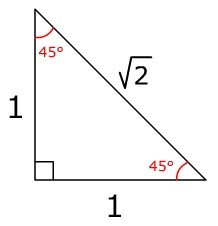A 45-45-90 triangle has side lengths in the ratio 1:1:√2 (the longest side is across from the largest angle).

Here, we can set up a proportion to solve for S:

• 1 / √2 = R / S [√3 and S/2 are the sides across from 45 degrees; 2 and R are the hypotenuses]
• 1*S = √2*R  [cross multiply]
• S = R√2

So, we can see that the side length S of the square is R√2.  Since √2 is approximately 1.41, we know that the side length S is about 41% larger than the radius R of the circle.

This makes sense, since S is the largest side of a square whose two other side lengths are R and R (meaning the length of the longest side is between R and 2R).

Alternately, the radius of the circle is the side length of the square divided by √2, or:

• R√2 = S
• R = S/√2  [divide both sides by √2]
• R = S√2/2  [rationalize the denominator]

Since the perimeter of a square is 4S (where S is the side length), then the perimeter of this square is 4S = 4(R√2) = (4√2)R, or approximately 5.657 times the length of the radius R of the circle.

Since the area of a square is S2 (length times width, which are both S), we have an area of S2= (R√2)2 = 2R2.  This is less than the area of the circle, πR2 (this makes sense, since the square is completely contained within the circle, so the square’s area should be smaller than the circle’s area).

(You can learn about squares vs circles in terms of perimeter, area, and other features here).

### Can A Pentagon Be Inscribed In A Circle?

A pentagon can be inscribed in a circle (along with other polygons, regular or not).  To find out the side length S of a pentagon inscribed in a circle of radius R, we would take steps similar to those above:

• Draw the diagram with a pentagon inscribed in a circle.
• Label the center of the circle (and pentagon) with a point C.
• Draw line segments from two adjacent vertices to the center point C (this gives us a triangle, which is 1/5 of the inscribed pentagon).  These line segments have a length of R (the radius of the circle).
• Fill in the angles for the triangle accordingly (for a pentagon, the central angle is 360/5 = 72 degrees, and so the other two angles are (180 – 72)/2 = 54 degrees.
• Use trigonometric functions (sine or cosine) to find the side length of the pentagon in terms of the radius R.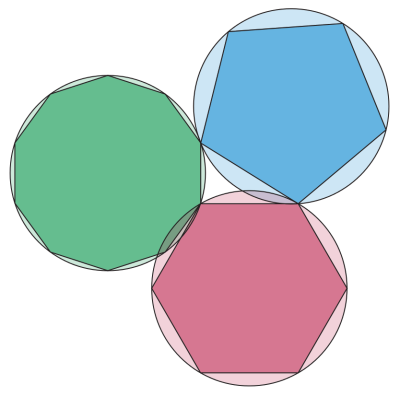Here we can see several inscribed regular polygons, including a pentagon (top right, in blue).

## Conclusion

Now you know how to work with various shapes inscribed in a circle to find perimeter or area, based on the radius of the circle.

I hope you found this article helpful.  If so, please share it with someone who can use the information.